## Measurement: Radius and Diameter of Circle

Practice Unlimited Questions

#### What is radius of a circle?

Any line drawn from the centre of a circle to the boundary or edge of the circle is a radius of the circle.

#### What is diameter of a circle?

Any straight line drawn from the boundary or edge of a circle to the opposite edge and which also passes through the center of the circle is a diameter of the circle. Diameter of a circle is always twice its radius.

#### Understanding a Circle

Take a circular paper disc, fold it in half and then unfold it.

The line of the fold that divides the circle into two halves is a diameter of the circle.
In the figure below, XY is a diameter.
Trace the outline of a CD, a round plate or a ring on paper and cut it along its edge to get a circular paper disc.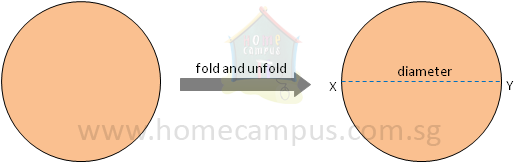Fold the paper disc in another way and you will get another diameter of the circle.
Name it ST as below.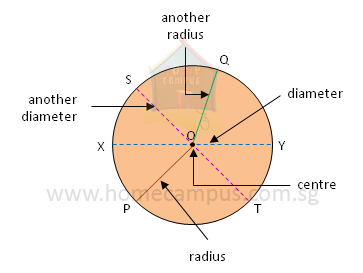Measure the lengths of the two diameters.
You will note that XY = ST.

Also, XY and ST meet at point O, which is known as the centre or center of the circle.

Any line drawn from the centre O, to the boundary or edge of the circle is a radius of the circle.
In the figure above, OP is a radius.
Measure the lengths of OP and OQ and you will notice that OP = OQ.
You will also note that the radius is equal to half the diameter.
OP  =
 1 2
XY
OP  =
 1 2
ST
Or,

#### 1. Find the radius of the circle below.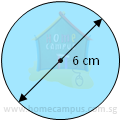Diameter  =  6 cm
Radius  =  6 cm  ÷  2  =  3 cm

#### 2. The diameter of a circle if 20 m. What is its radius?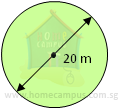Radius  =  20 m  ÷  2  =  10 m

#### 3. Find the diameter of the circle below.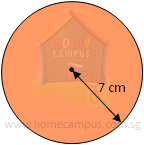Diameter  =  7 cm  ×  2  =  14 cm

#### 4. The radius of a circle is 15 m. Find its diameter.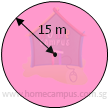Diameter  =  15 m  ×  2  =  30 m

#### GLOSSARY of terms related to circle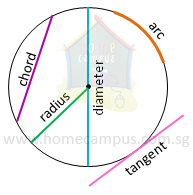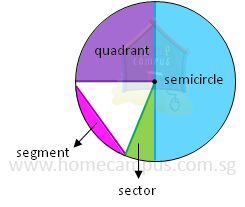Circumference: The perimeter of a circle.

Arc: A part of the circumference.

Chord: A line inside a circle that crosses the circumference at two points. A diameter is a chord that passes through the centre of the circle.

Tangent: A straight line that touches the circle at only one point.